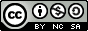## Wednesday, March 19, 2008

### Converting Decimal Values To Binary Without Using Unpack

Howdy,

Yesterday we took a look at using Unix or Linux Perl's unpack function to convert binary numbers to decimal. Today we're going to look at doing the opposite conversion ( decimal to binary ) and we're going to do it without using unpack :)

Actually, this script would be a whole lot shorter if we were to use the unpack function, but I wanted to highlight that Perl (and most scripting languages) can be used to achieve whatever ends you need met in any number of ways. Generally, your possibilities are limited only by your imagination (and the most basic rules ;)

To achieve the results we want from today's script, we're taking the input (the decimal number) and breaking it down into an array of binary values. The array that gets produced can be fed to yesterday's script to convert binary back to decimal just to verify, if you want :)

The process of converting the decimal number to a binary one in this script, breaks down (at its most basic level) to using the Perl exponential operator (**) to create a massive array of possible binary values and then using that same exponential math to determine whether each valid binary value is a 0 or a 1, and building the array (the actual answer) from that.

I hope these scripts serve as a halfway decent example of how you can do two almost identical things in two almost completely different ways, and, perhaps, as an incentive to write each of the two scripts (yesterday's and today's) in the manner of the other (Not necessary, and not necessarily fun, but a good exercise nonetheless ;)

Best wishes,`#!/usr/bin/perl## db - Convert decimal values to binary## 2008 - Mike Golvach - eggi@comcast.net## Creative Commons Attribution-Noncommercial-Share Alike 3.0 United States License#print "Decimal Value:\t";chomp (\$decimal=<stdin>);\$x = 100;\$total = "0";while (\$x != -1) { \$y = 2 ** \$x; \$total = \$total + \$y; --\$x;}\$a = 100;\$b = 0;\$decbin{\$a} = 2 ** \$a;while (\$a != -1) { if (\$decimal > \$total) {  print "Time to Upgrade, boy!\n";  exit; } \$decbin{\$a} = 2 ** \$a; if (\$decimal >= \$decbin{\$a}) {   \$decimal = \$decimal - \$decbin{\$a};  \$binarray[\$b] = 1;  --\$a;  \$b++; } else {  \$binarray[\$b] = 0;  --\$a;  \$b++; }} while (\$binarray != 1) {  \$tmp = shift(@binarray);  \$tmp = "0";  --\$x; }\$binarray = join("", @binarray);print "Binary Number:\t\$binarray\n";`